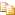﻿InsertRange Method (index, array, arrayIndex, count)AvalonEdit
InsertRange Method (index, array, arrayIndex, count)
NamespacesICSharpCode.AvalonEdit.UtilsRope<(Of <(<'T>)>)>InsertRange(Int32, array<T>[]()[][], Int32, Int32)
Inserts new elements into this rope. Runs in O(lg N + M), where N is the length of this rope and M is the number of new elements.Declaration Syntax
 C# Visual Basic Visual C++
```public void InsertRange(
int index,
T[] array,
int arrayIndex,
int count
)```
```Public Sub InsertRange ( _
index As Integer, _
array As T(), _
arrayIndex As Integer, _
count As Integer _
)```
```public:
void InsertRange(
int index,
array<T>^ array,
int arrayIndex,
int count
)```Parameters
index (Int32)
array (array<T>[]()[][])
arrayIndex (Int32)
count (Int32)Exceptions
ExceptionCondition
ArgumentNullExceptionnewElements is null.
ArgumentOutOfRangeExceptionindex or length is outside the valid range.

Assembly: ICSharpCode.AvalonEdit (Module: ICSharpCode.AvalonEdit.dll) Version: 5.0.0.4238